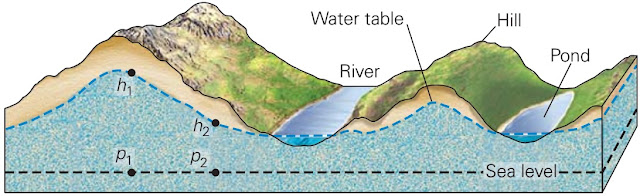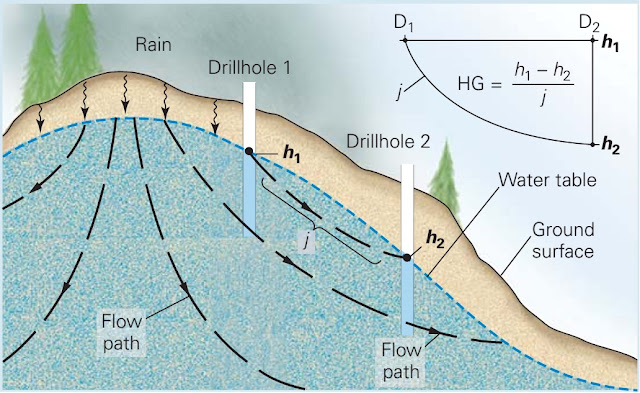## Groundwater Flow

What happens to groundwater over time? Does it just sit, unmoving, like the water in a stagnant puddle, or does it ﬂow and eventually ﬁnd its way back to the surface? Countless measurements conﬁrm that groundwater enjoys the latter fate groundwater indeed ﬂows, and in some cases it moves great distances underground. Let’s examine factors that drive groundwater ﬂow.
In the unsaturated zone the region between the ground surface and the water table water percolates straight down, like the water passing through a drip coffee maker, for this water moves only in response to the downward pull of gravity. But in the zone of saturation the region below the water table water ﬂow is more complex, for in addition to the downward pull of gravity, water responds to differences in pressure. Pressure can cause groundwater to ﬂow sideways, or even upward. (If you've ever watched water spray from a fountain, you've seen pressure pushing water upward.) Thus, to understand the nature of groundwater ﬂow, we must ﬁrst understand the origin of pressure in groundwater. For simplicity, we’ll consider only the case of groundwater in an  unconﬁned aquifer.The shape of water table beneath hilly topography.
Pressure in groundwater at a speciﬁc point underground is caused by the weight of all the overlying water from that point up to the water table. (The weight of overlying rock does not contribute to the pressure exerted on groundwater, for the contact points between mineral grains bear the rock’s weight.) Thus, a point at a greater depth below the water table feels more pressure than does a point at lesser depth. If the water table is horizontal, the pressure acting on an imaginary horizontal reference plane at a speciﬁed depth below the water table is the same everywhere. But if the water table is not horizontal, as shown in above, the pressure at points on a horizontal reference plane at depth changes with location. For example, the pressure acting at point p1, which lies below the hill in figure above, is greater than the pressure acting at point p2, which lies below the valley, even though both p1 and p2 are at the same elevation.
Both the elevation of a volume of groundwater and the pressure within the water provide energy that, if given the chance, will cause the water to ﬂow. Physicists refer to such stored energy as potential energy. The potential energy available to drive the ﬂow of a given volume of groundwater at a location is called the hydraulic head. To measure the hydraulic head at a point in an aquifer, hydrogeologists drill a vertical hole down to the point and then insert a pipe in the hole. The height above a reference elevation (for example, sea level) to which water rises in the pipe represents the hydraulic head water rises higher in the pipe where the head is higher. As a rule, groundwater ﬂows from regions where it has higher hydraulic head to regions where it has lower hydraulic head. This statement generally implies that groundwater regionally ﬂows from locations where the water table is higher to locations where the water table is lower.The flow of groundwater.
Hydrogeologists have calculated how hydraulic head changes with location underground, by taking into account both the effect of gravity and the effect of pressure. These calculations reveal that groundwater ﬂows along concave-up curved paths, as illustrated in cross section (figure above a, b). These curved paths eventually take groundwater from regions where the water table is high (under a hill) to regions where the water table is low (below a valley), but because of ﬂow-path shape,
some groundwater may ﬂow deep down into the crust along the ﬁrst part of its path and then may ﬂow back up, toward the ground surface, along the ﬁnal part of its path. The location where water enters the ground (where the ﬂow direction has a downward trajectory) is called the recharge area, and the location where groundwater ﬂows back up to the surface is called the discharge area (see figure above a).
Flowing water in an ocean current moves at up to 3 km per hour, and water in a steep river channel can reach speeds of up to 30 km per hour. In contrast, groundwater moves at less than a snail’s pace, between 0.01 and 1.4 m per day (about 4 to 500 m per year). Groundwater moves much more slowly than surface water, for two reasons. First, groundwater moves by percolating through a complex, crooked network of tiny conduits, so it must travel a much greater distance than it would if it could follow a straight path. Second, friction between groundwater and conduit walls slows down the water ﬂow.
Simplistically, the velocity of groundwater ﬂow depends on the slope of the water table and the permeability of the material through which the groundwater is ﬂowing. Thus, groundwater ﬂows faster through high-permeability rocks than it does through low-permeability rocks, and it ﬂows faster in regions where the water table has a steep slope than it does in regions where the water table has a gentle slope. For example, groundwater ﬂows relatively slowly (2 m per year) through a low-permeability aquifer under the Great Plains, but ﬂows relatively quickly (30 m per year) through a high-permeability aquifer under a steep hillslope. In detail, hydrogeologists use Darcy’s Law to determine ﬂow rates at a location.

### Darcy’s Law for Groundwater FlowThe level to which water rises in a drill hole is the hydraulic head (h). The hydraulic gradient (HG) is the difference in head divided by the length of the flow path.
The rate at which groundwater ﬂows at a given location depends on the permeability of the material containing the groundwater; groundwater ﬂows faster in a more permeable material than it does in a less permeable material. The rate also depends on the hydraulic gradient, the change in hydraulic head per unit of distance between two locations, as measured along the ﬂow path.
To calculate the hydraulic gradient, we divide the difference in hydraulic head between two points by the distance between the two points as measured along the ﬂow path. This can be written as a formula:
hydraulic gradient = h1 - h2/j
where h1 - h2 is the difference in head (given in meters or feet, because head can be represented as an elevation) between two points along the water table, and j is the distance between the two points as measured along the ﬂow path. A hydraulic gradient exists anywhere that the water table has a slope. Typically, the slope of the water table is so small that the path length is almost the same as the horizontal distance between two points. So, in general, the hydraulic gradient is roughly equivalent to the slope of the water table.
In 1856, a French engineer named Henry Darcy carried out a series of experiments designed to characterize factors that control the velocity at which groundwater ﬂows between two locations (1 and 2),  each of which has a different hydraulic head (h1 and h2). Darcy represented the velocity of ﬂow by a quantity called the discharge (Q), meaning the volume of water passing through an imaginary vertical plane perpendicular to the groundwater’s ﬂow path in a given time. He found that the discharge depends on the the hydraulic head (h1- h2); the area (A) of the imaginary plane through which the groundwater is passing; and a number called the hydraulic conductivity  (K). The hydraulic conductivity represents the ease with which a ﬂuid can ﬂow through a material. This, in turn, depends on many factors (such as the viscosity and density of the ﬂuid), but mostly it reﬂects the permeability of the material. The relationship that Darcy discovered, now known as Darcy’s law, can be written in the form of an equation as:
Q = KA(h1 - h2)/j
The equation states that if the hydraulic gradient increases, discharge increases, and that as conductivity increases, discharge increases. Put in simpler terms, the ﬂow rate of groundwater increases as the permeability increases and as the slope of the water table gets steeper.
Figures credited to Stephen Marshak.